## NETWORKDAYS.INTL Weekend working days

Returns the number of net working days between two provided days excluding specified weekend days and holidays.Google has introduced the Networkdays.intl function in December’ 2013

Let’s look at the syntax of the function

NETWORKDAYS.INTL(start_date, end_date, [weekend], [holidays])

Always remember when anything is square brackets [ ] that argument is optional, when ever you are using the fourth argument you have to use weekend argument otherwise you may get #Value error

the list of weekend are as follows: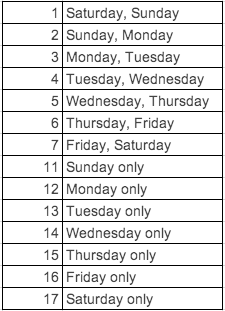Let’s try to understand the with an example.

Suppose we have dates in the cell B1 to B10 and we have holidays from Cell D2:D3 to find out the list working days between the given date we use the networkdays.intl function.

the formula in i used in the cell D5 is

=NETWORKDAYS.INTL(B1,B10,1,D2:D3)

in this example i have assumed that Saturday & Sundays are holidays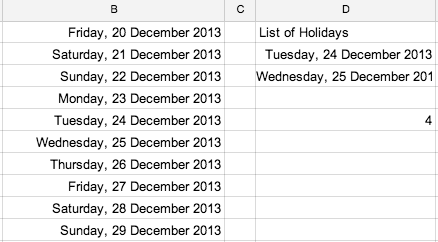the same thing can be written using binary

=NETWORKDAYS.INTL(B1,B10,”0000011″,D2:D3)

we will get the same results as 4

the advantage of writing with the binary form is suppose working days are only Friday, Saturday, Sunday and Monday something like a weekend market, we do not have such option in the weekend argument, so we have use the binary format. The formula will be

=NETWORKDAYS.INTL(B1,B10,”1000111″,D2:D3)

the result you will get is 7

## Query function with manipulation of dates

This post is in continuation of my earlier post on query function. for basic understand the query function have a look at the following link https://anandexcels.wordpress.com/2013/11/01/query-function-in-google-sheets/

https://anandexcels.wordpress.com/2013/11/07/single-column-manipulation-using-query-function/

https://anandexcels.wordpress.com/2013/11/08/query-function-refering-to-cell-value/

Now we will see how to manipulate Query function with DATES, manipulation of date is query function is tricky. some query does not understand the date because in the spreadsheets dates are treated as serial numbers and query understanding it as text. We have to trick the query function

(Q) Suppose we want list the data based on the certain dates referring to cell

we will continue to use the old example in my first query post

solution is as follows:

=QUERY(A:D;”select * where D>=date”””&text(F1,”yyyy-mm-dd”)&””” and D<=date”””&text(G1,”yyyy-mm-dd”)&””””)

where F1 is the start date and G1 is the end date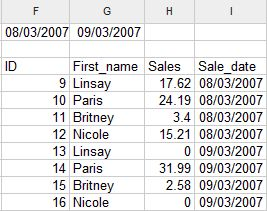Observations

(1) There are three double quote before text function and after text function

(2) we have used the text function to convert the numbers into text

(3) date has to be formatted in yyyy-mm-dd format only.

the link to spreadsheet is available in my first blogpost listed above

## Query function refering to cell value

This post is in continuation of my earlier post on query function. for basic understand the query function have a look at the following link https://anandexcels.wordpress.com/2013/11/01/query-function-in-google-sheets/

Now we look at the referring to cell value within query function so that query function will be more robust.

(Q) What is sales value during the period for Britney

we will continue use our old data set

(A) The formula we are using is

=QUERY(A:D;”select B, Sum(C) where B ='”&F1&”‘ group by B label Sum(C) ‘Sales'”;1)

the results will be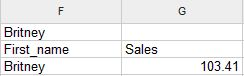where cell F1 contains the criteria in our example Britney

now we do not need to change the formula, if you change the cell value of F1 to from Britney to Paris the query function recalculates the values

the results will be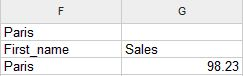Observations:

(1) Remember query function is case sensitive

(2) if you use paris instead of Paris you may get the null string without any error

(3) observe the syntax after where B =

(4) if Cell F1 is string you have use single-quote double-quote ampersand then F1 similarly after F1 also, this is a syntax you have to follow

## Single column manipulation using query function

This post is in continuation of my earlier post on query function. for basic understand the query function have a look at the following link https://anandexcels.wordpress.com/2013/11/01/query-function-in-google-sheets/

we will continue to use my earlier example only.

suppose if we want to count the First name and list them.

we can do this if we have multiple columns of data, suppose we have only one column we want to count as well as list them. you cannot do that using query function alone. we have to manipulate data using the Index function.

the formula for this is

=index(if({1,1},B2:B27))

this will create an array 2 X  26 on fly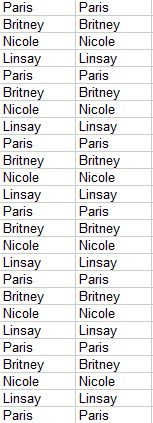Now we have two column of data we can use the query function to manipulate this

=query(index(if({1,1},B2:B27));”select Col1,count(Col2) where Col1 <>” group by Col1 label count(Col2) ‘Count’ “)

the result will be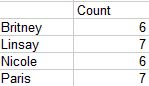Observations:

(1) We have not used the A, B instead we have used Col1 and Col2

(2) because the data is generated using the index function on fly

(3) we can create the copy of existing data using array constants

(4) the logic is within {1,1}

(5) you must use comma (in our example the data is single column), you should not use semi-colon

(6) comma is used for column manipulation and semi-colon is used for row manipulation

(7) this undocumented rules of array

Most versatile effective function and unique to Google Spreadsheets. I am documenting this function to understand it properly and for those who do not have programming background. I do not have previous experience with query. i will try to illustrate with examples.

If you have confusion and stuck some where please post your questions Google Docs forum at the following link https://productforums.google.com/forum/#!categories/docs/spreadsheets. There are experts in this area waiting for your questions.

Google Spreadsheet query is designed to be similar to SQL  with few exceptions. it is a subset of  SQL with a few feature of its own. if you are familiar with SQL it will be easy to learn.

The Syntax of the function is as follow:

DATA: it can be columns(A:C open ranges) of data you want to query, range of cells such as A1:C10, result of function such as importrange, index etc.,

QUERY: It is similar to SQL with small exceptions there is no FROM clause in the this since DATA itself is acting like a FROM clause.

HEADERS: If your data has headers in the row you can specify this here (suppose your first row has headers you can specify this as 1)

Notes:

1. column headers have to capital letter such A, B, C if you are picking up the raw data with in the same spreadsheet.

2. if you are using the array formulas to manipulate the data (Index, Filter, importrange to name a few) then column headers will Col1, Col2, Col3 etc. observe i have used C capital letter in Col1. this is syntax you have to follow this otherwise you will get a parse error

3. parse error:  an error of language resulting from code that does not conform to the syntax of the programming language; “syntax errors can be recognized at compilation time”

4. Data types: supports data types are string, number, boolean, date, datetime and timeof day. all values of the column will have a data type that matches the column type or a null value

5. The syntax of the query language is composed of the following clauses. Each clause starts with one or two keywords. All clauses are optional. Clauses are separated by spaces. The order of the clauses must be as follows:

 Clause Useage select Select which columns to return, and in what order, if omitted, all the table’s columns are returned, in their default order where Return only rows that match a condition. if omitted, all rows are returned. group by Aggregates values across rows pivot Transforms distinct values in columns into new column order by sorts rows by values in columns limit Limits the number of returned rows offset skips a given number of first rows label sets column labels format formats the values in certain columns using given formatting patterns options sets additional options

now let try to understand these clauses with an example

6. our data set like this

s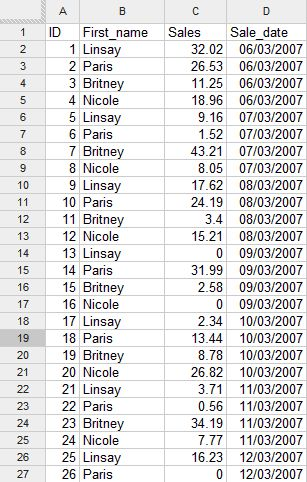7. try this formula

=QUERY(A:D;”select A,B”;1) the result will be as show in the below image

8.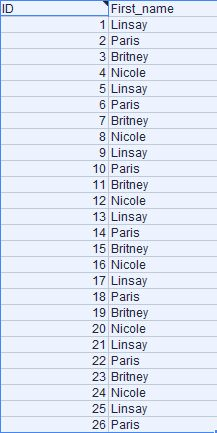We have some special keywords called functions, Functions are bits of code that perform an operation on a value or values. The first we will see is to perform a mathematical operation on a column. We will see Sum function which by totaling the values in a column designated by parentheses.

suppose we want to Sum Column C where the Column B is Nicole

=QUERY(A:D;”select B, Sum(C) where B = ‘Nicole’ group by B”;1)

9. the result will be

10.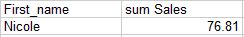Note:

(1) you have to use S capital in the Sum followed by column you want sum in parentheses

(2) whenever you are using the group by clause same column has to be selected in the select clause otherwise you may get value error

(3) condition you want the check in the column B has to be in single quote

this can be sorted using the order by clause

=QUERY(A:D;”select B, Sum(C) where B <>” group by B order by B asc”;1)

the result will be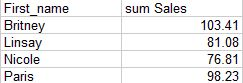Note:

(1) observe the column 1 has been sorted in ascending order

(2) to get the the descending order you can use the desc instead of asc

11. you can also sort the based on the result of the Sum(C)

=QUERY(A:D;”select B, Sum(C) where B <>” group by B order by Sum(C) desc”;1)

the results will be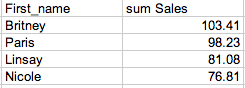Note:

(1) we have sorted data by descending order based on Sum of sales

(2) you have to follow the order of clause listed above

We can also limit the results top 3 or top 2 using the limit clause

=QUERY(A:D;”select B, Sum(C) where B <>” group by B order by Sum(C) desc limit 3″;1)We can also the name the column sum Sales as Top 3 sales

=QUERY(A:D;”select B, Sum(C) where B <>” group by B order by Sum(C) desc limit 3 label Sum(C) ‘Top 3 Sales'”;1)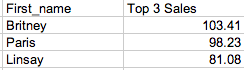We can also pivot the data based on date in the D column.

=QUERY(A:D;”select B, Sum(C) where B <>” group by B pivot D”;1)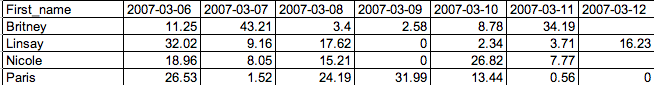Note:

(1) You might have observed the column D is not selected the select clause

(2) Date are formatted in yyyy-mm-dd format

(3) pivot is unique to google Sheets Query function

12. In the next blog post we will see how to manipulate data using the cell value

13. the link the spreadsheet is as follows:

## How much interest I can afford

The RATE function returns the interest rate of an investment, loan, or annuity based on a series of regular periodic cash flows.

The syntax of the rate function is

RATE(NPER, PMT, PV, FV, type, guess)

NPER:  The number of periods. NPER is a number value and must be greater than or equal to 0.

PMT: The payment made or amount received each period. payment is a number value. At each NPR, an amount received is a positive amount and an amount invested is a negative amount. It is simple monthly payment you are intended to make or receive

PV: (present value) The value of the initial investment, or the amount of the loan or annuity. present-value is a number value. At time 0, an amount received is a positive amount and an amount invested is a negative amount. In other words the amount of loan you intended to take

FV: An optional argument that represents the value of the investment or remaining cash value of the annuity (positive amount), or the remaining loan balance (negative amount), after the final payment. future-value is a number value. it could be ballon amount.

Type: Type (optional) defines whether the payment is due at the beginning (1) or the end (0) of a period

Guess: Guess (optional) determines the estimated value of the interest with iterative calculation. normally it is ignored

lets understand the function with an example:

I want to borrow 80,000 and make monthly payment for 10 years. The maximum monthly payment i can afford is 1,000. what is the maximum interest rate i can afford?

Solution:

In the above example

NPER: number of month here we have been provided with 10 year that means NPER is equal to 10 X 12 = 120

PMT: Payment for the period, in our example it is given as 1,000 per month

PV: present value. here the present value will be 80,000 which is amount i am borrowing now. we are inputting this value with – before it, because it will be cash outflow for the lender.

FV: it is an optional argument in out case we will put 0

Type: it can be either 1 or 0,  in our example we are putting 0 assuming the installment will be paid at the end of the period (i.e. month)

Guess: we can leave this argument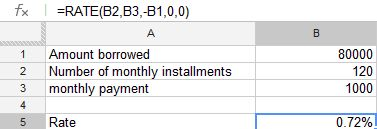the formula in cell B5 is =RATE(B2,B3,-B1,0,0)

I got the as 0.007241020100393 which i formatted using the % to convert it 0.72%

the above rate is period rate we have to convert this into annual rate

to convert this to annual rate we have multiplied it with 12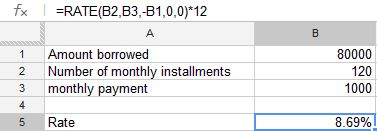for the formula in cell B5 is =RATE(B2,B3,-B1,0,0)*12

the solution to the problem is

i can afford up to 8.69% interest for my loan of 80,000/- for a period of 10 years

## IF FUNCTION

The IF function returns one of two values depending on whether a specified expression evaluates to a Boolean value of TRUE or FALSE.

IF(test, then_value, otherwise_value)

test:  A logical expression. test can contain anything as long as the expression can be evaluated as a Boolean. If the expression evaluates to a number, 0 is considered to be FALSE, and any other number is considered to be TRUE.

then_value:  The value returned if the expression is TRUE. if-true can contain any value type. If omitted (comma but no value), IF will return 0.

otherwise_value:  An optional argument specifying the value returned if the expression is FALSE. if-false can contain any value type. If omitted (comma but no value), IF will return 0. If entirely omitted (no comma after if-false) and if-expression evaluates to FALSE, IF will return FALSE.

Usage Notes

(1) If the Boolean value of test is TRUE, the function returns the then_value; otherwise it returns the otherwise_value. Both then_value and otherwise_value can contain additional IF functions (nested IF functions).

(2) the then_value & otherwise_value is optional argument. if left it still the IF function works. if the test results in 1/0 or TRUE/FALSE the result displayed by the function is TRUE/FALSE depending on the test value evaluated.

What is Boolean:

A Boolean value is one that can be expressed in only one of two values, as either TRUE or as FALSE. A FALSE value also has the value of 0. In this case, any other numeric value is considered TRUE. Boolean values are mostly used in comparisons.

Lets understand the IF function with an examples

Exp:1

If i order up to 500 units of the a product, I pay 10.00 per unit. if order from 501 through 1200 units, i pay 9.00 per unit. if i order from 1201 through 2000 units, i pay 8 per unit. if i order more than 2000 units, i pay 7 per unit. How can i write formula that expresses the purchase cost as a function of the number of units purchased?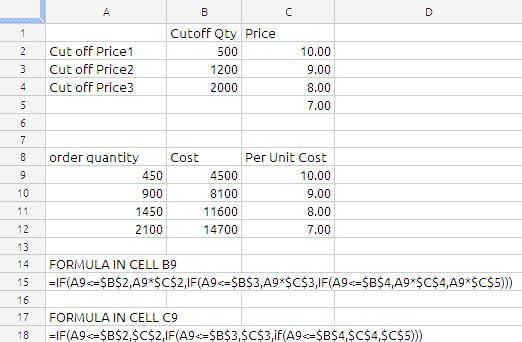we have used the nested IF conditions as shown in above image.

Exp 2:

If the value in Cell A1 is Red than value in the cell B1 will be 25 , if it is Blue then B1 has to be 50 and if A1 is Green then value in B1 has to be 75.

Solution: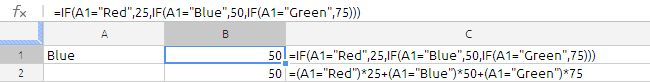in this case we have used two methods using IF function and using boolean algebra, Some time this type of situation using of boolean algebra will much more understandable and it is not constrained by IF function limitations.

This simple comparison will result in a value of TRUE if A2 has “Red” in it (just to be clear: the string of letters, not the background color). If there is anything else or even nothing in A2, then the result will be FALSE. True and False are actual values in the spreadsheet. If you were to enter this formula in some cell, you would see the word TRUE or the word FALSE in that cell, depending on what was in A2.

the spreadsheet is evaluated as shown below:

=(False)*25 + (True)*50 + (False)*75

= (0)*25+(1)*50+(0)*75

=0+50+0

=50

the other method of evaluating this is by use of CHOOSE function, the formula will be

=choose(MATCH(A1,F1:F3,0),25,50,75)

where F1 to F3 will hold Red,Blue, Green

we can also use the vlookup

The IF function has endless uses so we cannot document everything in one article. so i am concluding this article.